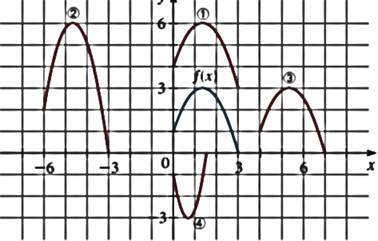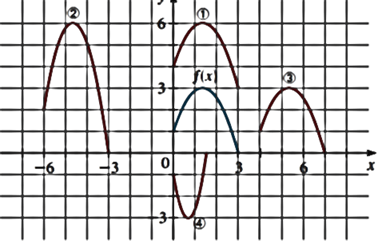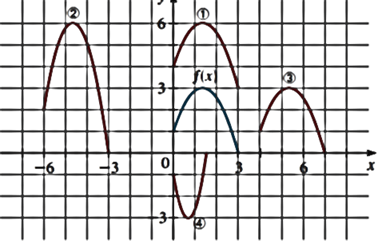# To match the equation of the function with its graph by obtaining the graph.### Precalculus: Mathematics for Calcu...

6th Edition
Stewart + 5 others
Publisher: Cengage Learning
ISBN: 9780840068071### Precalculus: Mathematics for Calcu...

6th Edition
Stewart + 5 others
Publisher: Cengage Learning
ISBN: 9780840068071

#### Solutions

Chapter 2.5, Problem 61E

a.

To determine

## To match the equation of the function with its graph by obtaining the graph.

Expert Solution

The equation y=f(x4) matches with the graph number 3 .

### Explanation of Solution

Given information:

The function is y=f(x)Use definition of horizontal shifting of the graph,

The graph of y=f(x4) is obtained by the graph of y=f(x) shifted 4 unit right, so from the above graph it is observed that graph number 3 matches with graph of equation y=f(x4) .

b.

To determine

### To match the equation of the function with its graph by obtaining the graph.

Expert Solution

The equation y=f(x)+3 matches with the graph number 1 .

### Explanation of Solution

Given information:

The function is y=f(x)Use definition ofvertical shifting of the graph,

The graph of y=f(x)+3 is obtained by the graph of y=f(x) shifted 3 unit upward, so from the above graph it is observed that graph number 1 matches with graph of equation y=f(x)+3 .

c.

To determine

### To match the equation of the function with its graph by obtaining the graph.

Expert Solution

The equation y=2f(x+6) matches with the graph number 2 .

### Explanation of Solution

Given information:

The function is y=f(x)Use definition of horizontal shifting and vertically stretching of the graph,

The graph of y=2f(x+6) is obtained by the graph of y=f(x) shifted 6 unit left then stretch the graph vertically by a factor of 2 , so from the above graph it is observed that graph number 2 matches with graph of equation y=2f(x+6) .

d.

To determine

### To match the equation of the function with its graph by obtaining the graph.

Expert Solution

The equation y=f(2x) matches with the graph number 4 .

### Explanation of Solution

Given information:

The function is y=f(x)Use definition of horizontal shrinking and reflecting of the graph,

The graph of y=f(2x) is obtained by the graph of y=f(x) shrink horizontally by a factor of 12 and then reflected in the x- axis, so from the above graph it is observed that graph number 4 matches with graph of this equation.

### Have a homework question?

Subscribe to bartleby learn! Ask subject matter experts 30 homework questions each month. Plus, you’ll have access to millions of step-by-step textbook answers!